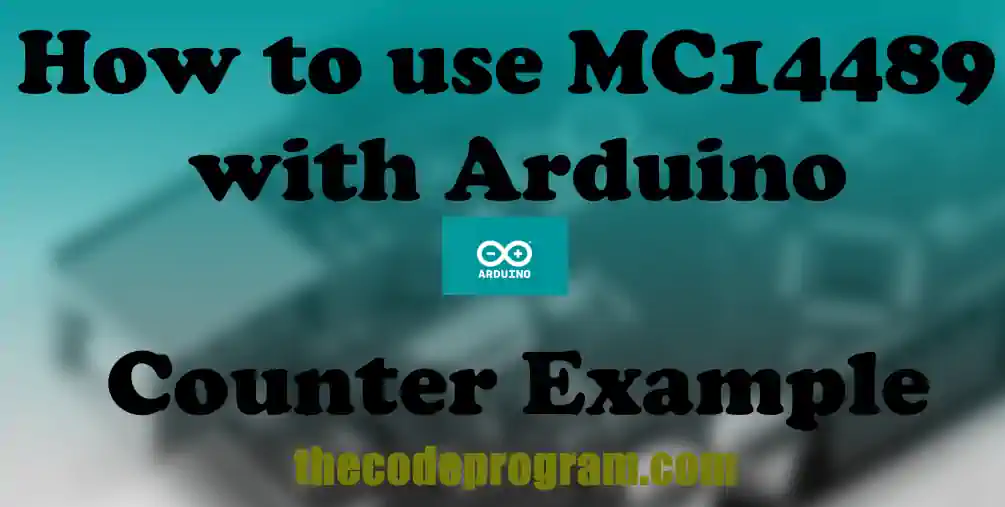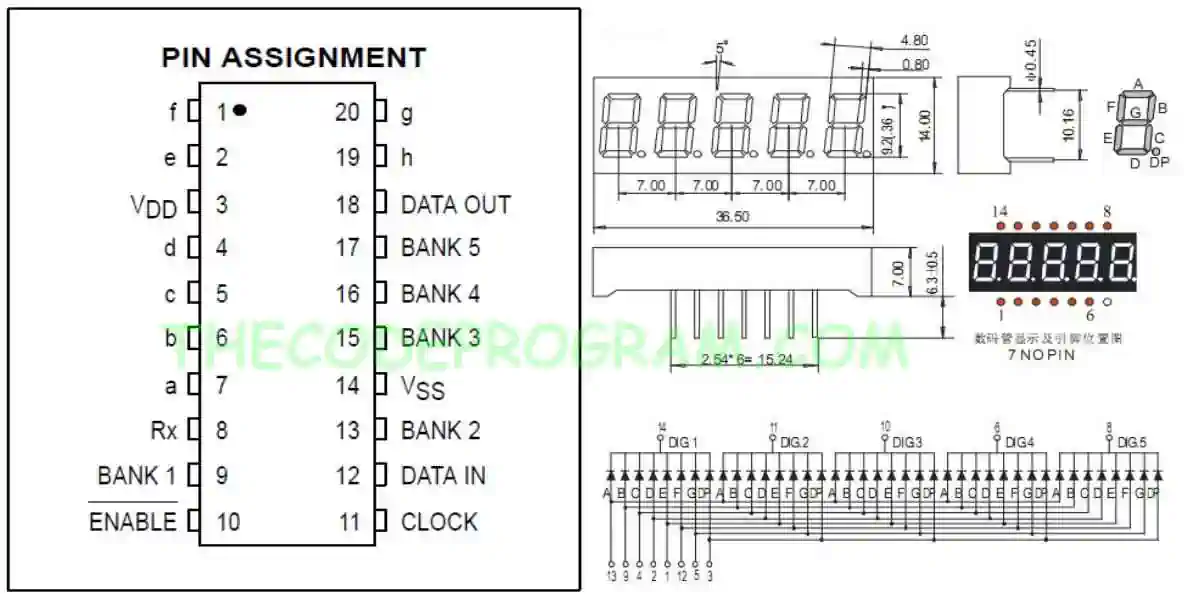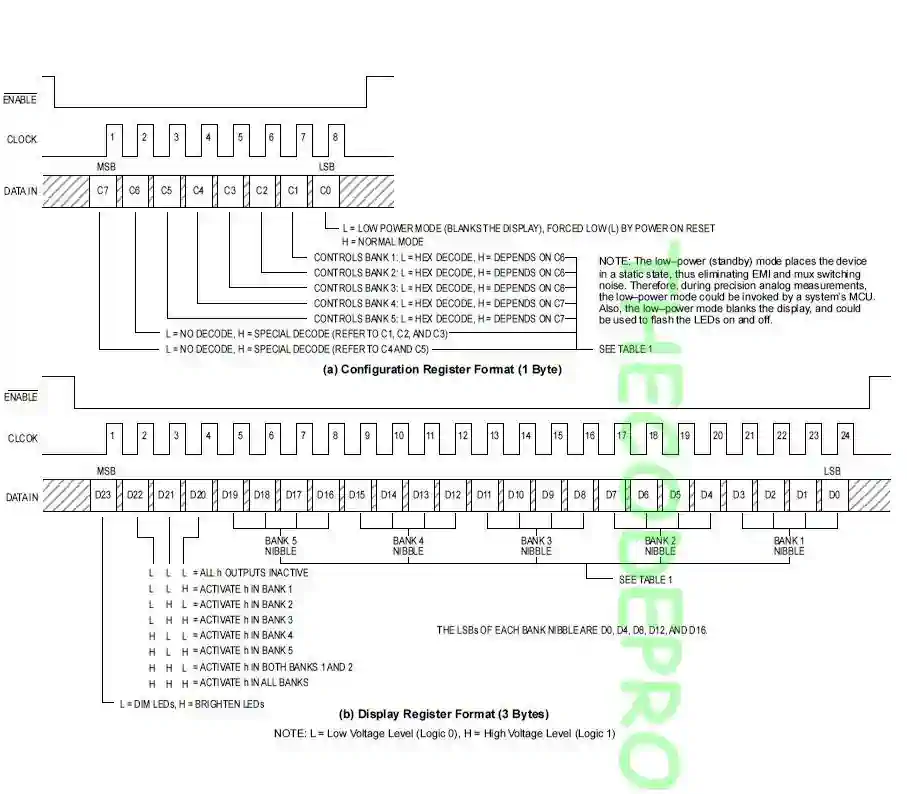## How to use MC14489 with Arduino Counter Example

Hello everyone, in this article we are going to talk about driving 5 Digit 7 Segment display with MC14489 with arduino. We are going to make an example on usage of MC14489 with Arduino.

Let's get started.

Firstly, What is MC14489
MC14489 is a LED driver which can be interfaced directly diodes. We can drive 7 segment displays or variations of this. LED's must be connected as common cathode logic. We can control maximum 5 digits with one MC14489 micro controller. MC14489 can be interfaced with SPI communication protocol. It accepts 24-bit data. We send all 24 digits numbers in 24bit . We can also send the configuration data with 8-bits.

Important Note : I always recommend to read the datasheet firstly.

Below image you can see pin configurations of MC14489 and 5x7 Segment LED Display.And do not forget to exemine the register data format of MC14489.In this example we are going to make a increasing counter one by one. Then we are going to show the current second on the display via MC14489.

Now let's start coding.

Firstly we need to declare our pins for communication. And we need to declare a 5 sized array to pass the digits to sending write.
``````
int data = 5;
int clk = 6;
int enable = 7;

byte digits;
``````

Now our essential method to send data to MC14489 driver. This method will write the data bit by bit via software SPI communication to MC14489. We will call this method from other functions for sending data to MC14489.

``````
void write_MC14489(byte digitData) {
int i=0;
int pinState;

digitalWrite(data, 0);
digitalWrite(clk, 0);

for (i=7; i>=0; i--)  {
digitalWrite(clk, 0);
if ( digitData & (1<<i) ) {
pinState= 1;
}
else {
pinState= 0;
}

digitalWrite(data, pinState);
digitalWrite(clk, 1);
digitalWrite(data, 0);
}

digitalWrite(clk, 0);
}
``````

And now we are going to parse the digits array to write values. We will send the datas as 8-bits. Because above write method is accepting 8 bit data. So I have sent all 24-bit value as three part. As you can see I have pulled down the enable pin then sent all 24-bit value and then pulled up back.

``````
void writeDigits(byte digits){
digitalWrite(enable, LOW);
write_MC14489(  (0xff << 4) | digits  ); // D23~D16
write_MC14489(  (digits << 4) | digits); // D15~D8
write_MC14489(  (digits << 4) | digits ); // D7~D0
digitalWrite(enable, HIGH);
}
``````
Below is our salad function. This function will count from 0 to 100000 at every second. It will parse every number and send them to MC14489 to show at display.
``````
void second_counter()
{
for(int i=0; i<100000; i++){

digits = i % 100000 /10000;
digits = i % 10000  /1000;
digits = i % 1000   /100;
digits = i % 100   / 10 ;
digits = i % 10     ;

writeDigits(digits);
delay(1000);
}

delay(10);
}
``````
Now we are ready. We are going to set the communication pins as OUTPUT and then we will enable the MC14489. At loop we will start the counter method.
``````
void setup()
{
pinMode(data, OUTPUT);
pinMode(enable, OUTPUT);
pinMode(clk, OUTPUT);

//Start the MC14489
digitalWrite(enable, LOW);
write_MC14489( 0x01 );
digitalWrite(enable, HIGH);
delay(10);
}

void loop()
{
second_counter();
}
``````

You can reach the example code on Github : https://github.com/thecodeprogram/Arduino_mc14489

Burak Hamdi TUFAN

11/07/2019

26/11/2019

05/04/2020